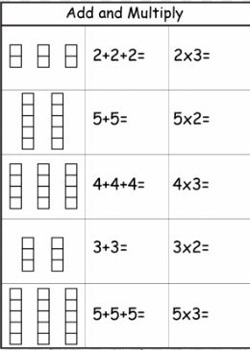Multiplication is defined as meaning that you have a certain number of groups of the same size. Then, it can be solved by repeated addition.

The result of multiplication is the total number (product) that would be obtained by combining several (multiplier) groups of similar size (multiplicand). The same result can be obtained by repeated addition. If we are combining 7 groups with 4 objects in each group, we could arrive at the same answer by addition. For example, 4+4+4+4+4+4+4=28 is equivalent to the multiplication equation 7*4=28.

The same result is also obtained by multiplication operation. Since 4 is being repeatedly added take 4 and multiply it with the number of groups which is 6.

So 4 × 6 = 24 (Read as 4 times 6 equals 24)

Here 4 and 6 care called as factors and the resulting number is called as product.The way multiplication is related to repeated addition can be explained in this way, i.e. multiplying a × b is the same as adding a repeatedly b number of times.

For example, for objects arranged in 4 rows and 5 columns (20 in all);

5 + 5 + 5 + 5 and 4 × 5 represents the total number of objects.

When you multiply, you add equal groups together to find the total.

Reference: AAA Math